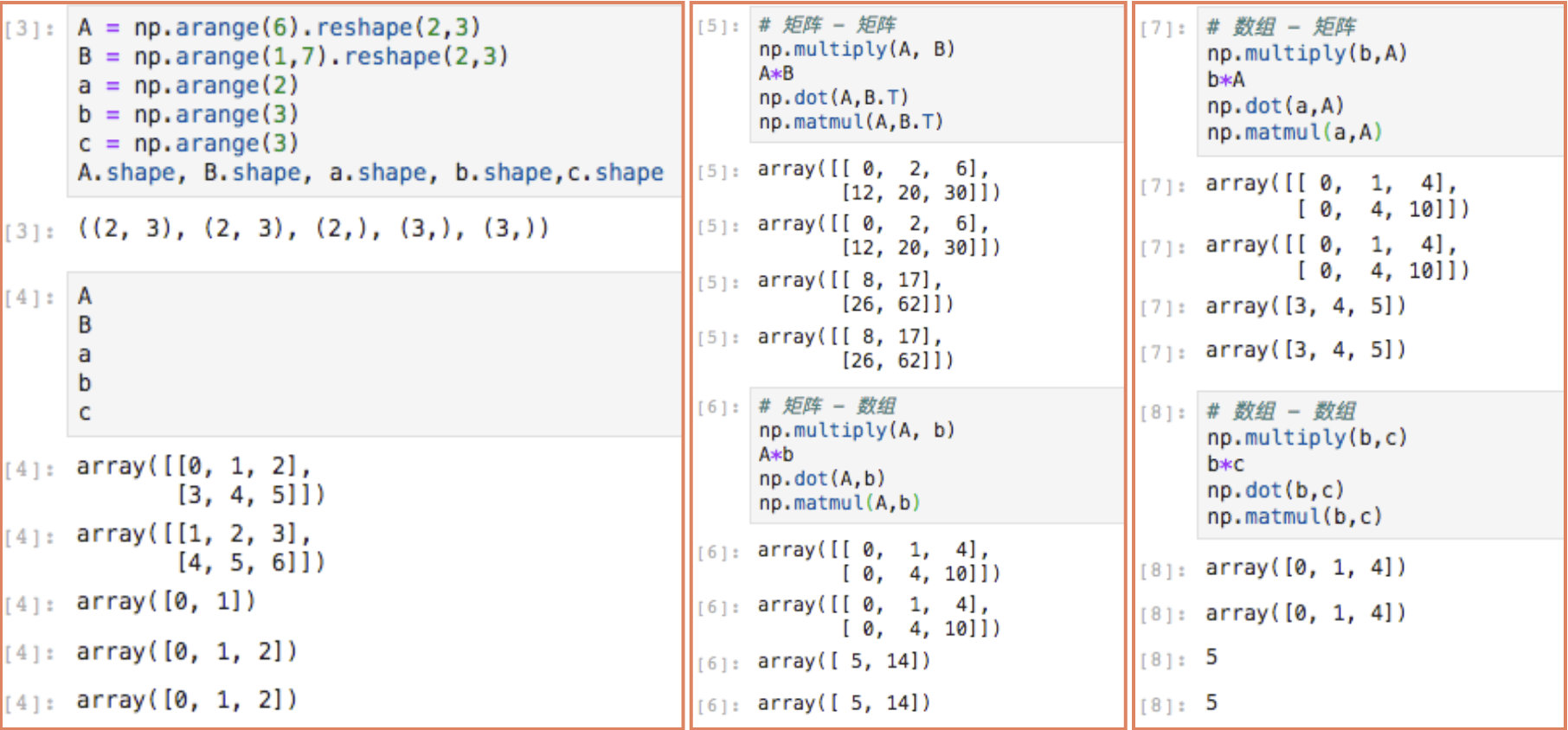• ## np.dot

千次阅读 2018-10-06 19:06:40
np.dot函数： 句法：np.dot(a,b) 解释：python中对a，b两个数组做点乘
np.dot函数：
句法：np.dot(a,b) 解释：python中对a，b两个数组做点乘
展开全文python numpy
• 同线性代数中矩阵乘法的定义： np.dot()np.dot(A, B)：对于二维矩阵，计算真正意义上的矩阵乘积，同线性代数中矩阵乘法的定义。对于一维矩阵，计算两者的内积。见如下Python代码：import numpy as np# 2-D array: 2...
Python中的几种矩阵乘法
1. 同线性代数中矩阵乘法的定义： np.dot()
np.dot(A, B)：对于二维矩阵，计算真正意义上的矩阵乘积，同线性代数中矩阵乘法的定义。对于一维矩阵，计算两者的内积。见如下Python代码：
import numpy as np

# 2-D array: 2 x 3
two_dim_matrix_one = np.array([[1, 2, 3], [4, 5, 6]])
# 2-D array: 3 x 2
two_dim_matrix_two = np.array([[1, 2], [3, 4], [5, 6]])

two_multi_res = np.dot(two_dim_matrix_one, two_dim_matrix_two)
print('two_multi_res: %s' %(two_multi_res))

# 1-D array
one_dim_vec_one = np.array([1, 2, 3])
one_dim_vec_two = np.array([4, 5, 6])
one_result_res = np.dot(one_dim_vec_one, one_dim_vec_two)
print('one_result_res: %s' %(one_result_res))

结果如下：
two_multi_res: [[22 28]
[49 64]]
one_result_res: 32
2. 对应元素相乘 element-wise product: np.multiply(), 或 *
在Python中，实现对应元素相乘，有2种方式，一个是np.multiply()，另外一个是*。见如下Python代码：
import numpy as np

# 2-D array: 2 x 3
two_dim_matrix_one = np.array([[1, 2, 3], [4, 5, 6]])
another_two_dim_matrix_one = np.array([[7, 8, 9], [4, 7, 1]])

# 对应元素相乘 element-wise product
element_wise = two_dim_matrix_one * another_two_dim_matrix_one
print('element wise product: %s' %(element_wise))

# 对应元素相乘 element-wise product
element_wise_2 = np.multiply(two_dim_matrix_one, another_two_dim_matrix_one)
print('element wise product: %s' % (element_wise_2))
结果如下：
element wise product: [[ 7 16 27]
[16 35  6]]
element wise product: [[ 7 16 27]
[16 35  6]]
展开全文python 矩阵乘法 multiply
• 1.二者都是矩阵乘法。 2.np.matmul中禁止矩阵与标量的乘法。...3.在矢量乘矢量的內积运算中，np.matmul与np.dot没有区别。 4.np.matmul中，多维的矩阵，将前n-2维视为后2维的元素后，进行乘法运算。 ...
1.二者都是矩阵乘法。 2.np.matmul中禁止矩阵与标量的乘法。 3.在矢量乘矢量的內积运算中，np.matmul与np.dot没有区别。 4.np.matmul中，多维的矩阵，将前n-2维视为后2维的元素后，进行乘法运算。
展开全文Python
• 总的来说，np.multiply()和*的作用是一样的，np.dot() 和np.matmul()作用是一样的。 1. np.multiply()函数 —— 星号（*）乘法运算 矩阵 - 矩阵：对应位置相乘 矩阵 - 数组：对应位置相乘 数组在后，行与数组相乘 ...
总的来说，np.multiply()和*的作用是一样的，np.dot() 和np.matmul()作用是一样的。
1. np.multiply()函数 —— 星号（*）乘法运算
矩阵 - 矩阵：对应位置相乘
矩阵 - 数组/数组 - 矩阵：对应位置相乘 数组在后或者在后，都是与矩阵的行对应相乘
数组 - 数组：对应位置相乘
数组和矩阵对应位置相乘，输出与相乘矩阵/数组的大小一致
2. np.dot()函数
对于秩为1的数组，执行对应位置相乘，然后再相加；
对于秩不为1的二维数组，执行矩阵乘法运算；超过二维的可以参考numpy库介绍。
输出大小：
矩阵与矩阵：遵循矩阵乘法应该有的大小矩阵 - 数组/数组 - 矩阵：数组在前，则数组维度应该与矩阵行数一致，结果维度为矩阵的列数；数组在后，则数组维度应该与矩阵列数一致，结果维度为矩阵的行数。

(

1

×

n

)

×

(

n

×

m

)

→

1

×

m

→

m

(1 \times n) \times (n \times m) \rightarrow 1 \times m \rightarrow m

，，

(

n

×

m

)

×

(

m

×

1

)

→

1

×

n

→

n

(n \times m) \times (m \times 1) \rightarrow 1 \times n \rightarrow n

数组 - 数组：常数
3. np.matmul()函数
np.matmul中禁止矩阵与标量的乘法。
在矢量乘矢量的內积运算中，np.matmul 与np.dot没有区别代码GitHub链接
展开全文• ## np.dot()

万次阅读 多人点赞 2019-11-17 09:07:09
x.dot(y) 等价于 np.dot(x,y) ———x是m*n 矩阵 ，y是n*m矩阵，则x.dot(y) 得到m*m矩阵。 如果处理的是一维数组，则得到的是两数组的內积。 如果处理的是二维数组（矩阵），则得到的是矩阵积 np.dot(x, y), 当x为...
• 1、np.dot() 2、@ 3、np.multiply() 4、*python 线性代数 numpy
• 这两天在看吴恩达深度学习作业的代码时，碰到了np.dot()、np.outer()、np.multiply()、*等四个函数，特此记录。 1、np.dot() 如果碰到的是秩为1的数组，那么执行的是对应位置的元素相乘再相加；如果遇到的是秩不为1...numpy outer multiply
• 3.在矢量乘矢量的內积运算中，np.matmul与np.dot没有区别。 4.np.matmul中，多维的矩阵，将前n-2维视为后2维的元素后，进行乘法运算。 >>>import numpy as np >>>a=np.array([1,2,3]) >>&...
• 矩阵乘法np.dot()及np.multipy()区别 1. 线性代数中矩阵乘法: np.dot() import numpy as np ​ # 2 x 3 matrix1 = np.array([[1, 2, 3], [4, 5, 6]]) ​ # 3 x 2 matrix2 = ...
• （1）：np.dot()如果碰到的是秩为1的数组，那么执行的是对应位置的元素相乘再相加;如果遇到的是秩不为1的数组，那么执行的是矩阵相乘。但是需要注意的是矩阵与矩阵相乘是秩为2，矩阵和向量相乘秩为1。 （2）：np....
• np.dot() performs a matrix-matrix or matrix-vector multiplication. This is different from np.multiply() and the * operator (which is equivalent to .* in Matlab/Octave), which performs an ...python
• Numpy中的矩阵向量乘法分别是np.dot(a,b)、np.multiply(a,b) 以及*，刚开始接触的时候比较模糊，于是自己整理了一下。先来介绍理论，然后再结合例子深入了解一下。 数组 矩阵 元素乘法 np.multiply(a,b) 或...numpy 矩阵
• ## np.dot()使用方法

万次阅读 多人点赞 2018-09-06 16:32:57
np.dot()函数主要有两个功能，向量点积和矩阵乘法，这里我就简单列举了三种最常用到的情况 1. np.dot(a, b), 其中a为一维的向量，b为一维的向量，当然这里a和b都是np.ndarray类型的, 此时因为是一维的所以是向量点...python
• 1、numpy.linalg ...2、np.dot() 对于矩阵：得到的结果就是矩阵相乘的结果 对于一维向量：得到的结果就是向量的内积 # 作用于矩阵 X = np.array([[1,2],[3,4]]) Y = np.array([[5,6],[7,8]]...
• 主要介绍了np.dot()函数的用法详解，文中通过示例代码介绍的非常详细，对大家的学习或者工作具有一定的参考学习价值，需要的朋友们下面随着小编来一起学习学习吧
• title: np.dot函数 date: 2019-11-15 00:56:38 categories: 机器学习 tags: 机器学习 np.dot()函数用法 Numpy中dot()函数主要功能有两个：向量点积和矩阵乘法。 格式：**x.dot(y) 等价于 np.dot(x,y) ———**x...
• 使用array时，运算符 * 用于计算数量积(点乘)，函数 dot() 用于计算矢量积...np.dot(A, B)：对于二维矩阵，计算真正意义上的矩阵乘积，同线性代数中矩阵乘法的定义。对于一维矩阵，计算两者的内积。 对应元素相...
• 矩阵乘法：np.dot(a,b) 或 np.matmul(a,b) 或 a.dot(b) 唯独注意：*，在 np.array 中重载为元素乘法，在 np.matrix 中重载为矩阵乘法! 对于** np.array **对象 >>> a array([[1, 2], [3, 4]]) 元素乘法...
• 针对向量： u.dot(v)=∣u∣∗∣v∣∗cosθu.dot(v)=|u|*|v|*cos \thetau.dot(v)=∣u∣∗∣v∣∗...可以用np.dot(u,v)，也可以写为u.dot(v) Caution: the * operator will perform an elementwise multiplication,...
• 在之前学习别人开源代码的时候，对于python中的*、np.dot()和np.multiply()具体结果产生了疑惑，遂去了解了一下相关说明，并实验了一下，结合别人的博客，这里进行总结 建议：当我们需要在python中进行像...python numpy
• x.dot(y) 等价于 np.dot(x,y) ———x是m*n 矩阵 ，y是n*m矩阵，则x.dot(y) 得到m*m矩阵。 如果处理的是一维数组，则得到的是两数组的內积。 如果处理的是二维数组（矩阵），则得到的是矩阵积 np.dot(x, y), 当x...
• 使用array时，运算符multiply、 * 用于分别计算两个数的相乘(1)，函数 dot() 同线性代数中矩阵乘法的定义（2）（对于秩为1的数组，执行对应位置相乘，然后再... 同线性代数中矩阵乘法的定义： np.dot()np.dot(A, B)...
• import tensorflow as tf import numpy as np x1 = ([[1, 2, 3], [1, 2, 3], [1, 2, 3]]) x2 = ([[2, 1, 1], [2, 1, 1], [2, 1, 1...y1 = np.dot(x1, x2) y2 = np.multiply(x1, x2) print('1、np.dot\n', y1) print...tensorflow numpy multiply matmul
• 目录np.multiply()函数作用代码示例np.dot()函数作用代码示例星号（*）函数作用代码示例 np.multiply() 函数作用 对应位置相乘 代码示例 import numpy as np Array1 = np.arange(0,4) #数组1 print("数组1:",Array1...
• In short， np.dot(a, b)就是一个乘法函数。 数和数相乘：若a和b都是数 np.dot(1, 2)=2 一维数组的内积 np.dot([1,2,3], [4,5,6])=[1,2,3]*[4,5,6]=1x4+2x5+3x6=32 矩阵的乘积 x = np.array([[1,2],[3,4]]) y = np...
• ## python的numpy中np.dot()详解

千次阅读 多人点赞 2019-06-12 17:39:55
dot函数是np中的矩阵乘法，假设 a,b为矩阵，a.dot(b) 等价于 np.dot(a,b) 。 a = np.array([2,3,4,5,6]) b = a.T print(a) print(b) print(np.dot(a,b)) print('**********') # 二维矩阵乘法 a = np.array([[1,1],......